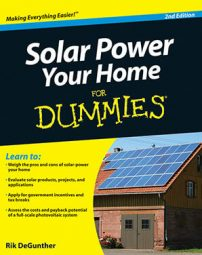##### Solar Power Your Home For DummiesIf you are installing solar power in your home, regardless of the application type, your first step is to assess your power needs. You can add up the power consumptions of individual appliances and other loads you need to run, but you also need to define how many loads will be running concurrently and at what times. Here are four specifications of interest:

• Total system capacity, measured in kilowatt-hours per day: This is the total amount of electricity you need over the course of a day.

• Peak instantaneous power output, measured in watts: You have a lot of control over this specification because you can run appliances at different times. For example, if your usual routine includes turning on the lights in the morning at the same time you turn on the coffee maker, you can change to a programmable coffee maker that has your coffee ready before you wake up. Or you can run your microwave oven only when all the lights are off, and so on.

• A measure of how often the system is used weekly (duty cycle): A weekend cabin used for two days a week has a duty cycle of 2/7, or approximately 28 percent. A system used every day has a duty cycle of 100 percent.

• How many hours of good sunlight a day you can expect: Estimating with much accuracy is difficult because sunlight depends a great deal on the weather. It also depends on the time of year in which you're interested in using your system.

The following table shows a sample load analysis chart for a weekend cabin. In this situation, the duty cycle is around 28 percent (two days per week), and the cabin is used only in the summer months, so the average expected sunlight per day is around eight hours.

Energy Consumption in a Weekend Cabin
AC Device Watts Hours/Day Watt-Hours/Day
Kitchen light 60 3 180
Family room light 120 4 480
TV 70 3 210
Coffeemaker 200 0.5 100
Microwave 900 0.15 135
Table fan 15 6 90
Refrigerator 20 24 480
Here's how to do some calculations from this chart:
• Determine the total daily energy needs (in kWh/day): Total the Watt-Hours/Day column and divide by 1,000.

• Figure the daily energy needs adjusted for inefficiency (10%): Multiply the total daily energy needs by 1.1.

• Figure the maximum instantaneous load: Decide which appliances will be on at the same time and add their power draws.

• Estimate the duty cycle: Divide the number of days per week the cabin is used by 7.

• Find the average kWh per day: Multiply the adjusted daily energy needs by the duty cycle.

The specifications that define the example system are the following (when you purchase a system, you need these numbers to tell system size and composition):

• Solar panel output: At eight hours of good sunlight per day, the example requires a 67.5-watt solar panel. (Take the average kWh per day of 0.54 and divide by 8 hours.) It's best to multiply this number by 1.3, just to be on the conservative side. This yields around 90 watts.

• Voltage output: In the example, it's 12VDC, but you can also use other voltages. (12VDC is the most common because it's what boats, cars, and RVs use.)

• Battery size: Calculate battery size, which is specified in terms of amp-hours (Ah). Most batteries are 12VDC, but other sizes are also available. For this example, assume you have a 12VDC system. Take the total adjusted kWh/day, multiply this by 1,000 to get kWh/day, and then divide this value by the battery voltage.

To get the best performance and economics out of a battery, it's best to over-spec the capacity by 100 percent, which yields around 300 Ah.

• Fuse size: Fuse size is determined by the maximum current draw. Take the maximum instantaneous load and divide by the voltage. For the example, this yields 100 amps:

This is a problem. Not only are currents higher than 30 amps dangerous, but the wire sizes also have to be very thick. Note that if you toss the microwave oven out of the picture, the maximum instantaneous power outage is only around 300 watts, which yields 25 amps. This number's practical. It's time to make a solar oven or get a camping stove that operates off of propane.

A basic 12VDC starter systems costs \$1,100 with battery and outputs 0.4 kWh per day (which isn't very much energy).